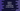# Python flatten a nested list or list of lists## Introduction :

Nested lists are lists holding other lists as its elements. These are also called lists of lists. If you want to flatten a nested list, you can easily do it by running a couple of for loops. In this tutorial, we will learn how to flat a nested list using a couple of different approaches.

## Method 1: using loops :

This is the easiest way to flat a list. We can iterate through the list elements using a loop and call the inner list items recursively to get the flat list. Example :

``````given_list = [1, 2, , [4, [5, 6,[7,]]]]
final_list = []

def flattenList(given_list):
for x in given_list:
if isinstance(x,list):
flattenList(x)
else:
final_list.append(x)

flattenList(given_list)
print(final_list)``````

Here, givenlist_ is the list of list. finallist_ is the final list i.e. the final flat list. We are iterating through the list elements one by one and if the current element is of type list, we are calling the same method recursively. Else, we are appending the element to the final flat list finallist_.

If you execute this program, it will print the below output :

``[1, 2, 3, 4, 5, 6, 7, 8]``

## Method 2: Using list comprehension :

With list comprehension and recursion, it becomes more easy to flat a list :

``````given_list = [1, 2, , [4, [5, 6, [7, ]]]]
final_list = []

def flattenList(given_list):
[final_list.append(x) if not isinstance(x, list)
else flattenList(x) for x in given_list]

flattenList(given_list)
print(final_list)``````

It will print the same output.

In these examples, we have flattened the list recursively because each list element can also hold a list and this depth can be infinite. If you know any other ways to solve it, drop one comment below.